# Doom Fire in LÖVE/lua

This weekend I read Fabien Sanglard’s post describing how the fire effect was implemented in Doom on the Playstation 1 and I figured this was a good chance to implement a small toy program in LÖVE.

Here’s an iterative tutorial, written for beginners, to demonstrate implementing this effect using Lua in the LÖVE environment. If you’re already experienced with Lua or programming in general then go read Fabien’s post. It’s much more succinct.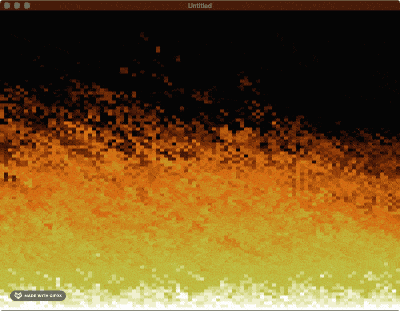## LÖVE program layout#

In LÖVE, a program consists of one or more `.lua` files in a folder. The most important file is `main.lua` and it needs to contain the following three functions:

``````function love.load()
end

function love.draw()
end

function love.update(dt)
end
``````

Make a folder with a file named `main.lua` with the above three functions, then open that folder in LÖVE and you’ll see… nothing. Well, not nothing. You’ll see a black window, which means you’ve successfully run a game that does nothing.

## Drawing a pixel grid#

LÖVE is optimized for modern hardware and a simpler development experience, so it operates mostly on objects and images instead of a raw pixel frame buffer. This is usually great, but we just want to set pixels to specific colors. So let’s start out by rendering a grid of rectangular “pixels”.

First off, we need to define a few constant numbers to use in a few places. Two of these will be named `WIDTH` and `HEIGHT` to represent the number of pixels to draw, and two more will be named `PWIDTH` and `PHEIGHT` to represent the size of each of our fake pixels.

### Global variables#

Define these constants at the top of `main.lua`:

``````WIDTH = 100
HEIGHT = 100
PWIDTH = love.graphics.getWidth() / WIDTH
PHEIGHT = love.graphics.getHeight() / HEIGHT
``````

We need one more global variable, but this one is something that will be modified a few times. Add an empty table named `pixels` under the constants.

``````pixels = {}
``````

### Generating color data#

Let’s fill out this table with some random colors to start. Do this in the `love.load()` function. This function will be run once when our game is started, so it’s good for loading or generating data.

``````function love.load()
for y = 1, HEIGHT do
pixels[y] = {}
for x = 1, WIDTH do
pixels[y][x] = {love.math.random(),love.math.random(),love.math.random()}
end
end
end
``````

From the top, this function runs a `for` loop from 1 to the height of our window in our fake pixels. It adds a new sub-table for each of these horizontal lines and then runs an inner `for` loop from 1 to the width of our window in our fake pixels. At this point, it can add one more sub-table representing the red/green/blue values of a random color for that pixel.

### Drawing pixels#

Finally, we can draw the pixels generated in the above loop. Fill out the `love.draw()` function, which is run on every frame and describes how to draw that frame.

``````function love.draw()
for y = 1, HEIGHT do
for x = 1, WIDTH do
color = pixels[y][x]
love.graphics.setColor(color, color, color, 1)
love.graphics.rectangle('fill', (x-1) * PWIDTH, (y-1) * PHEIGHT, PWIDTH, PHEIGHT)
end
end
end
``````

This function has the same nested `for` loops to loop over every pixel. The code then sets the current RGB color to the color in the `pixels` table at these coordinates. We get the red, green, and blue values out by indexing into the color at indices 1, 2, and 3. This might look strange if you’re used to other programming languages that start array offsets at index 0. Lua starts with index 1.

After setting the current drawing color, the code draws a pixel, or rather, a rectangle that is used to represent a fake pixel. The code gets the real coordinates on the window by multiplying the x,y coordinates by the pixel size (after first subtracting 1 to get back to a 0-based index). Then it draws a rectangle of size `PWIDTH * PHEIGHT`.

It’s important to note that the x,y coordinates of the window start at (0,0) in the top left and extend downward and to the right. This is different from graph coordinates in math, but is typical in graphics programming.

### Result#

Complete code
``````WIDTH = 100
HEIGHT = 100
PWIDTH = love.graphics.getWidth() / WIDTH
PHEIGHT = love.graphics.getHeight() / HEIGHT
pixels = {}

function love.load()
for y = 1, HEIGHT do
pixels[y] = {}
for x = 1, WIDTH do
pixels[y][x] = {love.math.random(),love.math.random(),love.math.random()}
end
end
end

function love.draw()
for y = 1, HEIGHT do
for x = 1, WIDTH do
color = pixels[y][x]
love.graphics.setColor(color, color, color, 1)
love.graphics.rectangle('fill', (x-1) * PWIDTH, (y-1) * PHEIGHT, PWIDTH, PHEIGHT)
end
end
end

function love.update(dt)
end
``````

We don’t need to fill out `love.update()` yet, because the program isn’t going to change the pixels right now. Open your folder in LÖVE to run it and check out the crazy pattern.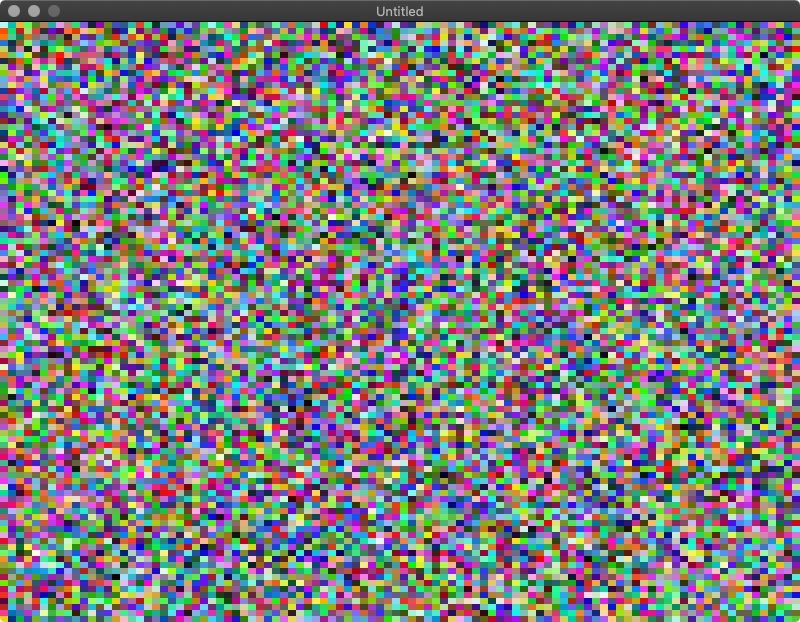## Color palette#

Random colors look interesting, but we need to use a palette for the fire effect. A palette is a set of colors that our program will choose from for each pixel.

### Palette table#

Up at the top of `main.lua`, add the palette table. Each of these entries is a sub-table of red, green, and blue values from 0-255. These colors range from black at the start, through a range of reds, and finally white at the end.

``````palette = {
{7,7,7},
{28,8,8},
{43,17,9},
{65,19,11},
{80,28,13},
{95,36,16},
{110,38,18},
{133,47,22},
{148,55,25},
{163,70,30},
{178,79,33},
{185,80,34},
{208,89,39},
{208,96,40},
{208,96,40},
{201,102,41},
{202,109,45},
{195,115,45},
{196,123,47},
{197,130,49},
{198,138,53},
{190,137,53},
{191,145,54},
{192,152,60},
{186,160,61},
{186,160,61},
{187,167,67},
{187,167,67},
{189,175,73},
{182,175,72},
{183,182,74},
{183,182,79},
{207,206,124},
{223,223,166},
{239,239,203},
{255,255,255}
}
``````

### Switching pixels to palette values#

Let’s make some changes to how pixels are stored so that the code references the RGB values in the palette instead of a different RGB value per pixel.

In `love.load()`, change

``````pixels[y][x] = {love.math.random(),love.math.random(),love.math.random()}
``````

to

``````pixels[y][x] = math.floor(love.math.random() * (table.maxn(palette) - 1) + 1)
``````

`math.random()` generates a decimal value from 0 to 1, and `table.maxn()` gives the maximum index of the `palette` table (36 because there are 36 palette entries). We want a random value from 1 to 36, so we can multiply the random number by 35 to get a number from 0 to 35, then add 1.

There’s one more issue, which is that LÖVE expects RGB values to be decimals from 0 to 1, but the palette table has whole numbers from 0-255. Add one more chunk of code to `love.load()` to convert our palette values to the correct range.

``````for i, v in ipairs(palette) do
palette[i] = {v/255, v/255, v/255}
end
``````

This is a different form of a `for` loop. Inside this `for` loop, `i` refers to the current index out of all of the indices in `palette`, and `v` refers to the current value.

### Drawing with the palette#

Now we need to draw pixels by looking up their color from the palette. Replace the drawing code inside the loops in `love.draw()` with:

``````color = pixels[y][x]
love.graphics.setColor(palette[color], palette[color], palette[color], 1)
love.graphics.rectangle('fill', (x-1) * PWIDTH, (y-1) * PHEIGHT, PWIDTH, PHEIGHT)
``````

This is almost the same as before, but with a level of indirection to look up the RGB value from the palette.

### Result#

Complete code
``````WIDTH = 100
HEIGHT = 100
PWIDTH = love.graphics.getWidth() / WIDTH
PHEIGHT = love.graphics.getHeight() / HEIGHT
pixels = {}

palette = {
{7,7,7},
{28,8,8},
{43,17,9},
{65,19,11},
{80,28,13},
{95,36,16},
{110,38,18},
{133,47,22},
{148,55,25},
{163,70,30},
{178,79,33},
{185,80,34},
{208,89,39},
{208,96,40},
{208,96,40},
{201,102,41},
{202,109,45},
{195,115,45},
{196,123,47},
{197,130,49},
{198,138,53},
{190,137,53},
{191,145,54},
{192,152,60},
{186,160,61},
{186,160,61},
{187,167,67},
{187,167,67},
{189,175,73},
{182,175,72},
{183,182,74},
{183,182,79},
{207,206,124},
{223,223,166},
{239,239,203},
{255,255,255}
}

function love.load()
for y = 0, HEIGHT do
pixels[y] = {}
for x = 0, WIDTH do
pixels[y][x] = math.floor(love.math.random() * (table.maxn(palette) - 1) + 1)
end
end

for i, v in ipairs(palette) do
-- convert palette from "bytes" to floats
palette[i] = {v/255, v/255, v/255}
end
end

function love.draw()
for y = 1, HEIGHT do
for x = 1, WIDTH do
color = pixels[y][x]
love.graphics.setColor(palette[color], palette[color], palette[color], 1)
love.graphics.rectangle('fill', (x-1) * PWIDTH, (y-1) * PHEIGHT, PWIDTH, PHEIGHT)
end
end
end

function love.update(dt)
end
``````

With these changes we get similar random noise, but with colors selected from our black-red-white palette.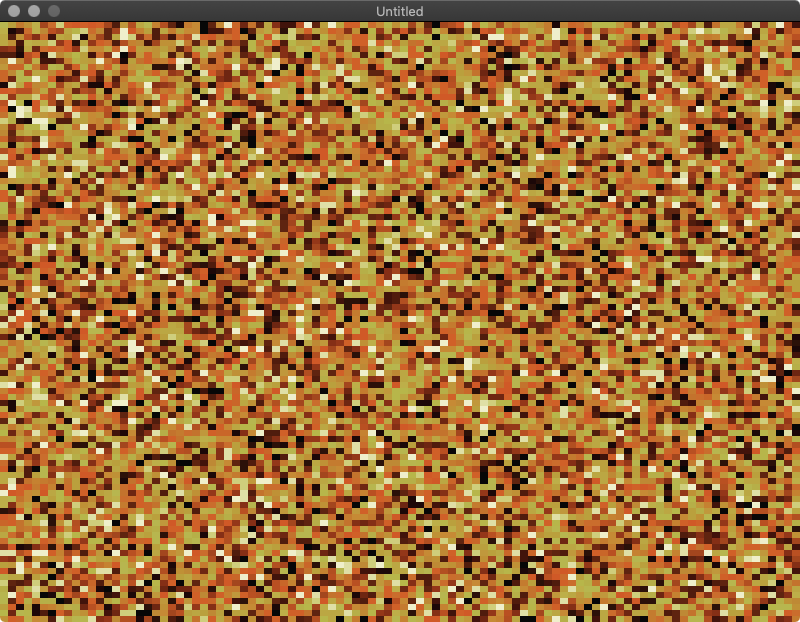## Basic fire#

Alright, enough with the random noise. Let’s do something that looks more like fire.

### Starting state#

First, let’s correct the starting state of our pixels. The screen needs to start all black, except for one white line at the bottom. That white line will serve as the generator of the fire.

In `love.load()` change

``````pixels[y][x] = math.floor(love.math.random() * (table.maxn(palette) - 1) + 1)
``````

to the simpler

``````pixels[y][x] = 1
``````

This sets all pixels to the first value of our palette, black.

Then add this chunk of code to the bottom of `love.load()`:

``````for x = 1, WIDTH do
pixels[HEIGHT][x] = table.maxn(palette)
end
``````

This sets the last (bottom) line of pixels to white, the last value of our palette.

### Propagate fire#

Now we can implement the `doFire()` and `spreadFire()` methods that Fabien described in his blog.

`doFire()` is simple. It just iterates over every pixel, except for the top line, and runs `spreadFire()` on that pixel.

``````function doFire()
for y = 2, HEIGHT do
for x = 1, WIDTH do
spreadFire(x, y)
end
end
end
``````

`spreadFire()` is also surprisingly simple. It just propagates the current pixel up to the pixel above it, subtracting one from the palette index so that the new pixel moves one step along the palette.

``````function spreadFire(x,y)
pixels[y-1][x] = math.max(1, pixels[y][x] - 1)
end
``````

Then just call `doFire()` from `love.update()`. This will update the state of the fire on every frame.

``````function love.update(dt)
doFire()
end
``````

### Result#

Complete code
``````WIDTH = 100
HEIGHT = 100
PWIDTH = love.graphics.getWidth() / WIDTH
PHEIGHT = love.graphics.getHeight() / HEIGHT
pixels = {}

palette = {
{7,7,7},
{28,8,8},
{43,17,9},
{65,19,11},
{80,28,13},
{95,36,16},
{110,38,18},
{133,47,22},
{148,55,25},
{163,70,30},
{178,79,33},
{185,80,34},
{208,89,39},
{208,96,40},
{208,96,40},
{201,102,41},
{202,109,45},
{195,115,45},
{196,123,47},
{197,130,49},
{198,138,53},
{190,137,53},
{191,145,54},
{192,152,60},
{186,160,61},
{186,160,61},
{187,167,67},
{187,167,67},
{189,175,73},
{182,175,72},
{183,182,74},
{183,182,79},
{207,206,124},
{223,223,166},
{239,239,203},
{255,255,255}
}

function love.load()
for y = 1, HEIGHT do
pixels[y] = {}
for x = 1, WIDTH do
-- init screen to black
pixels[y][x] = 1
end
end

for x = 1, WIDTH do
-- bottom pixels start at white
pixels[HEIGHT][x] = table.maxn(palette)
end

for i, v in ipairs(palette) do
-- convert palette from "bytes" to floats
palette[i] = {v/255, v/255, v/255}
end
end

function love.draw()
for y = 1, HEIGHT do
for x = 1, WIDTH do
color = pixels[y][x]
love.graphics.setColor(palette[color], palette[color], palette[color], 1)
love.graphics.rectangle('fill', (x-1) * PWIDTH, (y-1) * PHEIGHT - 1, PWIDTH, PHEIGHT)
end
end
end

function doFire()
for y = 2, HEIGHT do
for x = 1, WIDTH do
spreadFire(x, y)
end
end
end

function spreadFire(x,y)
pixels[y-1][x] = math.max(1, pixels[y][x] - 1)
end

function love.update(dt)
doFire()
end
``````

This gives us a nice, though static, gradient along our palette.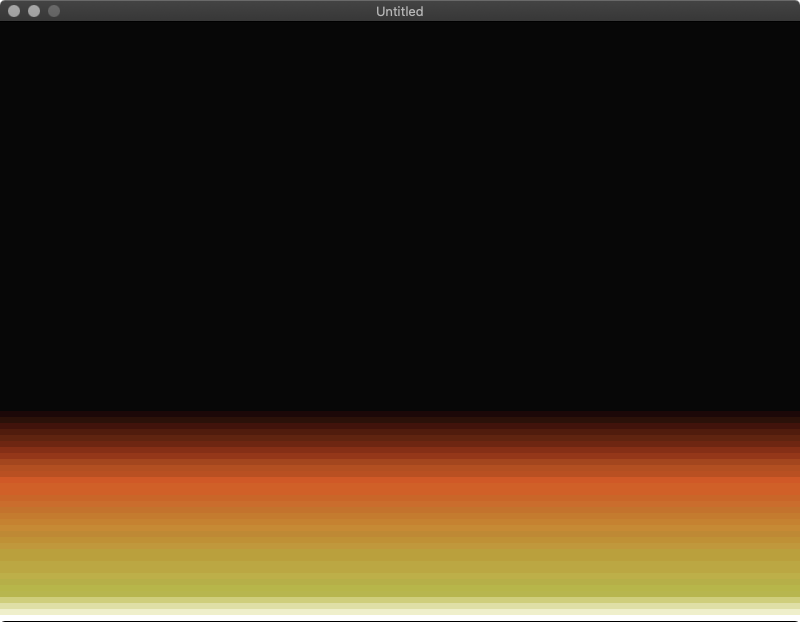## Simple randomness#

A perfect static fire is boring, so let’s add some randomness. We’ll start out by just adding randomness along the Y-axis.

Modify `spreadFire()`:

``````function spreadFire(x,y)
rand = math.floor(math.random() + 0.5)
pixels[y-1][x] = math.max(1, pixels[y][x] - rand)
end
``````

First this code randomly gets either 0 or 1. Adding 0.5 and then flooring the number effectively rounds it to the nearest whole number, since Lua doesn’t have a built-in function to round numbers.

Then `spreadFire()` subtracts this random 0 or 1 from the pixel value, using `math.max()` to ensure it never goes below the minimum valid color index of 1.

### Result#

Complete code
``````WIDTH = 100
HEIGHT = 100
PWIDTH = love.graphics.getWidth() / WIDTH
PHEIGHT = love.graphics.getHeight() / HEIGHT
pixels = {}

palette = {
{7,7,7},
{28,8,8},
{43,17,9},
{65,19,11},
{80,28,13},
{95,36,16},
{110,38,18},
{133,47,22},
{148,55,25},
{163,70,30},
{178,79,33},
{185,80,34},
{208,89,39},
{208,96,40},
{208,96,40},
{201,102,41},
{202,109,45},
{195,115,45},
{196,123,47},
{197,130,49},
{198,138,53},
{190,137,53},
{191,145,54},
{192,152,60},
{186,160,61},
{186,160,61},
{187,167,67},
{187,167,67},
{189,175,73},
{182,175,72},
{183,182,74},
{183,182,79},
{207,206,124},
{223,223,166},
{239,239,203},
{255,255,255}
}

function love.load()
for y = 1, HEIGHT do
pixels[y] = {}
for x = 1, WIDTH do
-- init screen to black
pixels[y][x] = 1
end
end

for x = 1, WIDTH do
-- bottom pixels start at white
pixels[HEIGHT][x] = table.maxn(palette)
end

for i, v in ipairs(palette) do
-- convert palette from "bytes" to floats
palette[i] = {v/255, v/255, v/255}
end
end

function love.draw()
for y = 1, HEIGHT do
for x = 1, WIDTH do
color = pixels[y][x]
love.graphics.setColor(palette[color], palette[color], palette[color], 1)
love.graphics.rectangle('fill', (x-1) * PWIDTH, (y-1) * PHEIGHT - 1, PWIDTH, PHEIGHT)
end
end
end

function doFire()
for y = 2, HEIGHT do
for x = 1, WIDTH do
spreadFire(x, y)
end
end
end

function spreadFire(x,y)
rand = math.floor(math.random() + 0.5)
pixels[y-1][x] = math.max(1, pixels[y][x] - rand)
end

function love.update(dt)
doFire()
end
``````

This finally starts to look fire-like.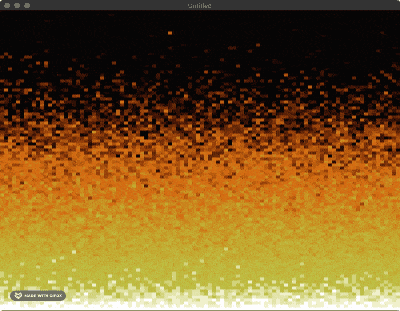## Better randomness#

With a small change to `spreadFire()` we can make the fire more realistic by adding randomness along the X-axis.

First of all, we’re going to be doing bitwise operations, which Lua doesn’t support natively. Pull in a library to do these operations by adding this line to the very top of `main.lua`:

``````local bit = require("bit")
``````

Now modify `spreadFire()`:

``````function spreadFire(x,y)
rand = math.floor(math.random() * 3 + 0.5)
pixels[y-1][x - rand + 1] = math.max(1, pixels[y][x] - bit.band(1, rand))
end
``````

This code now gets a random number from 0-3. It then randomly changes the destination X coordinate by -2 to +1 and sets the palette index to the value of the pixel below it minus a random value of 0 or 1. The 0 or 1 comes from performing a bitwise AND on the random 0-3 number to get the lowest bit.

### Result#

Complete code
``````local bit = require("bit")

WIDTH = 100
HEIGHT = 100
PWIDTH = love.graphics.getWidth() / WIDTH
PHEIGHT = love.graphics.getHeight() / HEIGHT
pixels = {}

palette = {
{7,7,7},
{28,8,8},
{43,17,9},
{65,19,11},
{80,28,13},
{95,36,16},
{110,38,18},
{133,47,22},
{148,55,25},
{163,70,30},
{178,79,33},
{185,80,34},
{208,89,39},
{208,96,40},
{208,96,40},
{201,102,41},
{202,109,45},
{195,115,45},
{196,123,47},
{197,130,49},
{198,138,53},
{190,137,53},
{191,145,54},
{192,152,60},
{186,160,61},
{186,160,61},
{187,167,67},
{187,167,67},
{189,175,73},
{182,175,72},
{183,182,74},
{183,182,79},
{207,206,124},
{223,223,166},
{239,239,203},
{255,255,255}
}

function love.load()
for y = 1, HEIGHT do
pixels[y] = {}
for x = 1, WIDTH do
-- init screen to black
pixels[y][x] = 1
end
end

for x = 1, WIDTH do
-- bottom pixels start at white
pixels[HEIGHT][x] = table.maxn(palette)
end

for i, v in ipairs(palette) do
-- convert palette from "bytes" to floats
palette[i] = {v/255, v/255, v/255}
end
end

function love.draw()
for y = 1, HEIGHT do
for x = 1, WIDTH do
color = pixels[y][x]
love.graphics.setColor(palette[color], palette[color], palette[color], 1)
love.graphics.rectangle('fill', (x-1) * PWIDTH, (y-1) * PHEIGHT - 1, PWIDTH, PHEIGHT)
end
end
end

function doFire()
for y = 2, HEIGHT do
for x = 1, WIDTH do
spreadFire(x, y)
end
end
end

function spreadFire(x,y)
rand = math.floor(math.random() * 3 + 0.5)
pixels[y-1][x - rand + 1] = math.max(1, pixels[y][x] - bit.band(1, rand))
end

function love.update(dt)
doFire()
end
``````

Looks great! Here’s some nice pixelated fire, from only a small amount of lua.## Extra credit: Turning the fire on and off#

I mentioned earlier than the line of white pixels at the bottom of the window acts like the generator of the fire. So what happens if we turn off the generator?

Try setting the bottom line to black when you press the space bar.

``````function love.update(dt)
if love.keyboard.isDown('space')
then
for x = 1, WIDTH do
-- turn off generator line by setting it to black
pixels[HEIGHT][x] = 1
end
else
for x = 1, WIDTH do
-- turn generator line back on
pixels[HEIGHT][x] = table.maxn(palette)
end
end

doFire()
end
``````

### Result#

Complete code
``````local bit = require("bit")

WIDTH = 100
HEIGHT = 100
PWIDTH = love.graphics.getWidth() / WIDTH
PHEIGHT = love.graphics.getHeight() / HEIGHT
pixels = {}

palette = {
{7,7,7},
{28,8,8},
{43,17,9},
{65,19,11},
{80,28,13},
{95,36,16},
{110,38,18},
{133,47,22},
{148,55,25},
{163,70,30},
{178,79,33},
{185,80,34},
{208,89,39},
{208,96,40},
{208,96,40},
{201,102,41},
{202,109,45},
{195,115,45},
{196,123,47},
{197,130,49},
{198,138,53},
{190,137,53},
{191,145,54},
{192,152,60},
{186,160,61},
{186,160,61},
{187,167,67},
{187,167,67},
{189,175,73},
{182,175,72},
{183,182,74},
{183,182,79},
{207,206,124},
{223,223,166},
{239,239,203},
{255,255,255}
}

function love.load()
for y = 1, HEIGHT do
pixels[y] = {}
for x = 1, WIDTH do
-- init screen to black
pixels[y][x] = 1
end
end

for x = 1, WIDTH do
-- bottom pixels start at white
pixels[HEIGHT][x] = table.maxn(palette)
end

for i, v in ipairs(palette) do
-- convert palette from "bytes" to floats
palette[i] = {v/255, v/255, v/255}
end
end

function love.draw()
for y = 1, HEIGHT do
for x = 1, WIDTH do
color = pixels[y][x]
love.graphics.setColor(palette[color], palette[color], palette[color], 1)
love.graphics.rectangle('fill', (x-1) * PWIDTH, (y-1) * PHEIGHT - 1, PWIDTH, PHEIGHT)
end
end
end

function doFire()
for y = 2, HEIGHT do
for x = 1, WIDTH do
spreadFire(x, y)
end
end
end

function spreadFire(x,y)
rand = math.floor(math.random() * 3 + 0.5)
pixels[y-1][x - rand + 1] = math.max(1, pixels[y][x] - bit.band(1, rand))
end

function love.update(dt)
if love.keyboard.isDown('space')
then
for x = 1, WIDTH do
-- turn off generator line
pixels[HEIGHT][x] = 1
end
else
for x = 1, WIDTH do
-- turn generator line back on
pixels[HEIGHT][x] = table.maxn(palette)
end
end

doFire()
end
``````

Pixely but realistic looking fire that can turn on and off at the press of a button!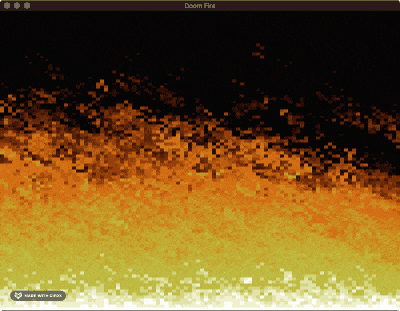## Exercises for the reader#

Here are a couple of interesting tweaks you can try to implement to check your understanding of the code. See if you can complete each one by changing only a single line of code.

• Try changing the fire color to green or blue
• Try making the fire shorter

Thanks to Jackie Loven for editing.

© 2021 Dylan Garrett